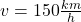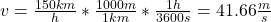## Calcular la velocidad media de un carro que recorrio 390 kilometros en 150 kilometros por hora. a) expresa el resultado en km/h b)expresa el

Question

Calcular la velocidad media de un carro que recorrio 390 kilometros en 150 kilometros por hora. a) expresa el resultado en km/h b)expresa el resultado en m/s porfa es urgente

in progress 0
5 months 2021-09-05T10:11:35+00:00 1 Answers 0 views 0

a) v = 150 km/h

b) v = 41.66 m/s

Explanation:

a) The average velocity in km/h is given by the statement in the exercise:b) To calculate the average velocity in m/s you need a to do the following conversion:hence, you have that the average velocity of the car is 150km/h = 41.66 m/s